# Fraction calculator

The calculator performs basic and advanced operations with fractions, expressions with fractions combined with integers, decimals, and mixed numbers. It also shows detailed step-by-step information about the fraction calculation procedure. Solve problems with two, three, or more fractions and numbers in one expression.

## Result:

### 3/7 + 1/8 = 31/56 ≅ 0.5535714

Spelled result in words is thirty-one fifty-sixths.

### How do you solve fractions step by step?

1. Add: 3/7 + 1/8 = 3 · 8/7 · 8 + 1 · 7/8 · 7 = 24/56 + 7/56 = 24 + 7/56 = 31/56
For adding, subtracting, and comparing fractions, it is suitable to adjust both fractions to a common (equal, identical) denominator. The common denominator you can calculate as the least common multiple of both denominators - LCM(7, 8) = 56. In practice, it is enough to find the common denominator (not necessarily the lowest) by multiplying the denominators: 7 × 8 = 56. In the next intermediate step, the fraction result cannot be further simplified by canceling.
In words - three sevenths plus one eighth = thirty-one fifty-sixths.

#### Rules for expressions with fractions:

Fractions - use the slash “/” between the numerator and denominator, i.e., for five-hundredths, enter 5/100. If you are using mixed numbers, be sure to leave a single space between the whole and fraction part.
The slash separates the numerator (number above a fraction line) and denominator (number below).

Mixed numerals (mixed fractions or mixed numbers) write as non-zero integer separated by one space and fraction i.e., 1 2/3 (having the same sign). An example of a negative mixed fraction: -5 1/2.
Because slash is both signs for fraction line and division, we recommended use colon (:) as the operator of division fractions i.e., 1/2 : 3.

Decimals (decimal numbers) enter with a decimal point . and they are automatically converted to fractions - i.e. 1.45.

The colon : and slash / is the symbol of division. Can be used to divide mixed numbers 1 2/3 : 4 3/8 or can be used for write complex fractions i.e. 1/2 : 1/3.
An asterisk * or × is the symbol for multiplication.
Plus + is addition, minus sign - is subtraction and ()[] is mathematical parentheses.
The exponentiation/power symbol is ^ - for example: (7/8-4/5)^2 = (7/8-4/5)2

#### Examples:

subtracting fractions: 2/3 - 1/2
multiplying fractions: 7/8 * 3/9
dividing Fractions: 1/2 : 3/4
exponentiation of fraction: 3/5^3
fractional exponents: 16 ^ 1/2
adding fractions and mixed numbers: 8/5 + 6 2/7
dividing integer and fraction: 5 ÷ 1/2
complex fractions: 5/8 : 2 2/3
decimal to fraction: 0.625
Fraction to Decimal: 1/4
Fraction to Percent: 1/8 %
comparing fractions: 1/4 2/3
multiplying a fraction by a whole number: 6 * 3/4
square root of a fraction: sqrt(1/16)
reducing or simplifying the fraction (simplification) - dividing the numerator and denominator of a fraction by the same non-zero number - equivalent fraction: 4/22
expression with brackets: 1/3 * (1/2 - 3 3/8)
compound fraction: 3/4 of 5/7
fractions multiple: 2/3 of 3/5
divide to find the quotient: 3/5 ÷ 2/3

The calculator follows well-known rules for order of operations. The most common mnemonics for remembering this order of operations are:
PEMDAS - Parentheses, Exponents, Multiplication, Division, Addition, Subtraction.
BEDMAS - Brackets, Exponents, Division, Multiplication, Addition, Subtraction
BODMAS - Brackets, Of or Order, Division, Multiplication, Addition, Subtraction.
GEMDAS - Grouping Symbols - brackets (){}, Exponents, Multiplication, Division, Addition, Subtraction.
Be careful, always do multiplication and division before addition and subtraction. Some operators (+ and -) and (* and /) has the same priority and then must evaluate from left to right.

## Fractions in word problems:7 is added to the sum of 4/5 and 6/7
• Samuel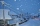Samuel has 1/3 of a bag of rice and Isabella has a 1/2 bag of rice. What fraction of are bag of rice do they have altogether?
• Cups of flour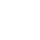Jade was baking cupcakes for her class. She has 4 5/4 cups of flour.  For one batch, she used 1 2/3 cups of flour.  On another batch, she used 7/9 of a cup of flour.  How much flour does Jade have left after making the two batches of cupcakes?
• A dumpA dump truck bought 1/3 of a ton of rock on the first trip, 1/2 of a ton on the second trip, and 4/5 of a ton on the third trip. What was the total weight of the rock?
• Patel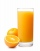Patel squeezed oranges so that his family could have fresh-squeezed juice for breakfast. He squeezed 4/17 cups from the first orange, 3/10 cups from the second orange, StartFraction 9 over 20 EPeter bought 1/2 a pound of chocolate at rocky mountain chocolate factory. Later he went to the sweet shoppie and he bought 6/9 of a pound more chocolate. How much chocolate did he buy that day?
• Berry SmoothieRory has 5/8 cup of milk. How much milk does she have left after she doubles the recipe of the smoothie? Berry Smoothie: 2 cups strawberries 1 cup blueberries 1/4 cup milk 1 tbsp (tablespoon) sugar 1/2 tsp (teaspoon) lemon juice 1/8 tsp (teaspoon) vanilla
• How many 3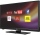How many hours the Andersons watched TV in all Wednesday 3/1 hr Thursdays 2/3 hr Friday 4/5 hr Saturday 3/4 hr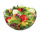We need two tenths kg of a carrot, one tenth of peas and three tenths of of tomatoes to make salad. Express the fraction of the weight of the vegetables to be salad. Convert the result to grams.What is 4 1/2+2/7-213/14?Ann ate a third of a pizza and then another quater. Total part of pizza eaten by Ann and how much pizza is left?Jacobs mom bought two whole pizzas. He ate 2/10 of the pizza and his dad ate 1 1/5. How much is left.The sum of two rational numbers is (-2). If one of them is 3/5, find the other.